Technical Article

# Use LTspice to Understand the LM741 OpAmp: Part 2

November 22, 2015 by Tim Youngblood

## How an op amp works with LTspice simulation. We left off last time finishing up how the input stage of an op-amp works. The next important stage is made up by transistors Q3, Q4, Q5, Q6, and Q7.

### How op-amps work: Part 2 of 2

We left off last time finishing up how the input stage of an op-amp works. The next important stage is made up by transistors Q3,Q5, Q6, Q7, and Q8. The emitters of the differential input (see Part 1) are connected to the emitters of Q5 and Q6, this provides for level shifting, which is required for voltage swing and DC level input at the second stage. The current­ mirror load is made up of Q3­,Q6, R1, and ­R2. Let’s walk through this a little more.

$$I_{CQ7} = I_{CQ8}$$

$$I = I_{CQ5} = I_{CQ6} = I_{CQ7} = I_{CQ8}$$

$$I_{CQ3} = \frac{2 I}{\beta} + \frac{V_{BEQ8} + I R_{2}}{R_{3}} = \frac{2 I}{\beta} + \frac{V_{T} \ln (\frac{I}{I_{S}}) + I R_{2}}{R_{3}}$$

Hopefully, these equations shed a little more light on the signal path and general functioning of the 741 op-amp thus far...I assure you, the input stage we completed in Part 1 is the most complicated part of this circuit.

Recall the input stage: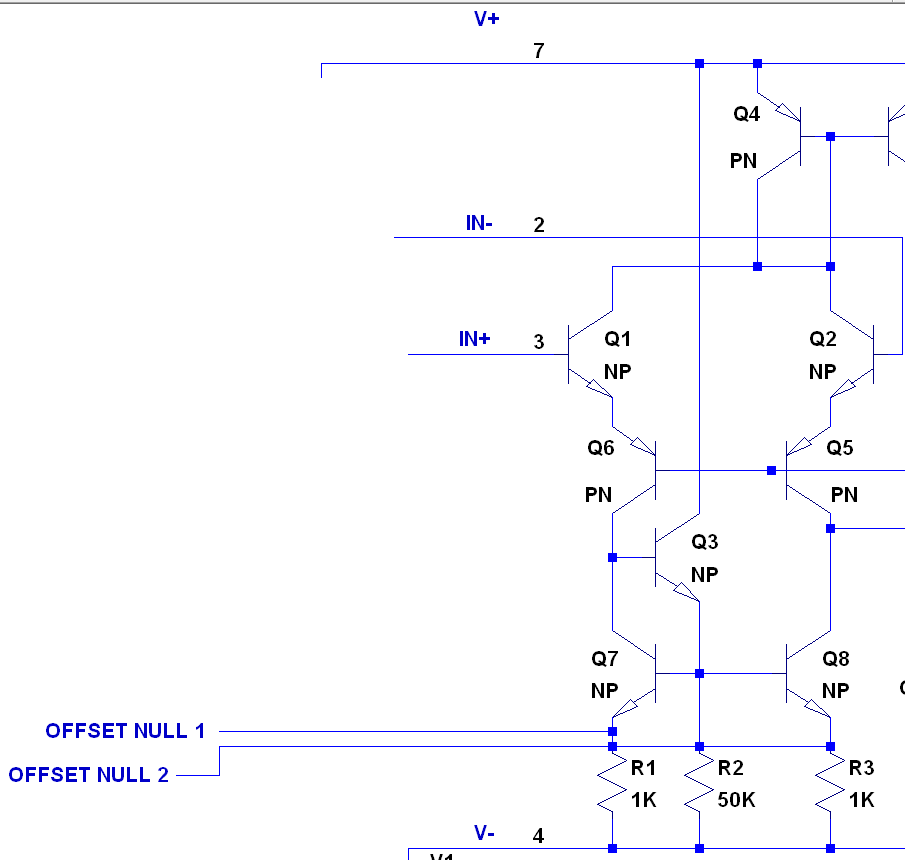##### Figure 1

One more feature you may have noticed is the offset null 1 and 2. In simulation this is not necessary to tweak, but in real life it will be necessary to include a pot between offset null 1 and 2. Why? Well, when there is no difference between the two inputs (Q1 and Q2) you may get a nonzero output. This is due to transistor and resistor mismatches...nothing's perfect! To balance the mismatches and obtain a zero output when there is no difference in input, put a pot between offset null 1 and 2 and trim it until your output looks good. To read further on the mathematics about this, Texas Instruments has already worked out some good math about the subject as seen here.

Now this is great and all: we have a pretty solid input stage, things are looking up, we kind of understand what’s going on...But wait! We have a differential input and the 741 op-amp only has one output. How do we convert the differential input to a single ended signal? Whoa, wait a second, what? Oh don’t worry, the creator of the 741 (David Fullagar) already thought this out for us. The active load (Q3,­Q8, R1,­R2) create a modified Wilson current mirror. Its purpose is to take the differential input current and make it a single-ended signal without the 50% loss of converting from two signals to one. Follow? It gets a little fuzzy, I know. A small signal differential current in Q6 versus Q5 looks summed at the base of Q16. Study the active load schematic closely...It might take some time to absorb this concept, but you'll get it.

Get it? Got it? Good.

Ok, now we finally can move on from that input stage. The single-ended signal is fed to Q16. Q16 and Q15 create a Darlington configuration. That is, the current amplified by the first transistor Q16 is subsequently amplified again by Q15. This creates a high current gain( $$\beta \beta$$). Q17 exists as a form of negative feedback to prevent saturation. If it gets too high, Q17 turns on and diverts the base current going into Q16 to ground, thus stabilizing the configuration. This amplifier uses the output side of the current mirror created between Q10 and Q11; it is an active load. Active loads lead to significant voltage gain because if the active load were perfect the voltage gain would be infinite if you worked out the thevenin equivalent resistance for a current source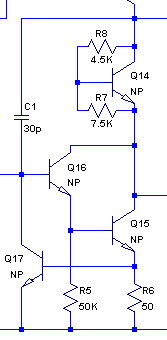##### Figure 2

In figure 2,  Q14 provides a voltage level shift. The level shifter stage is included to ensure that there is no DC offset in the output signal. DC offset arises from the turn on voltages of the transistors throughout the circuit. As a exercise run the simulation with and without Q14 noting the differences between the two plots.

Finally we arrive at the output stage! The output stage is composed of Q18, Q20 and Q19. The output stage is a Class AB push pull emitter follower amplifier, go look that up if you're not familiar with the concept. Q18 provides output current limiting much like we saw with Q17. That’s it! Go have fun with the simulation. Play around with it and I think it will all start coming together.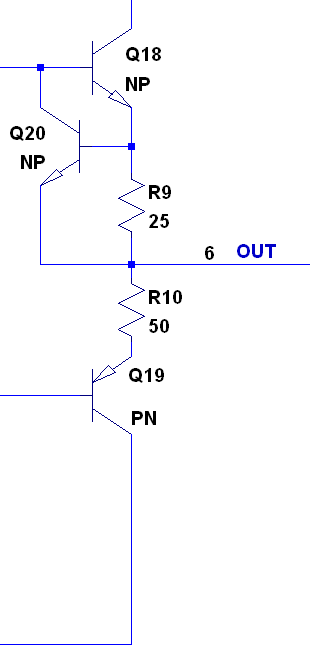Simulating an inverting amplifier with unity gain (click to enlarge)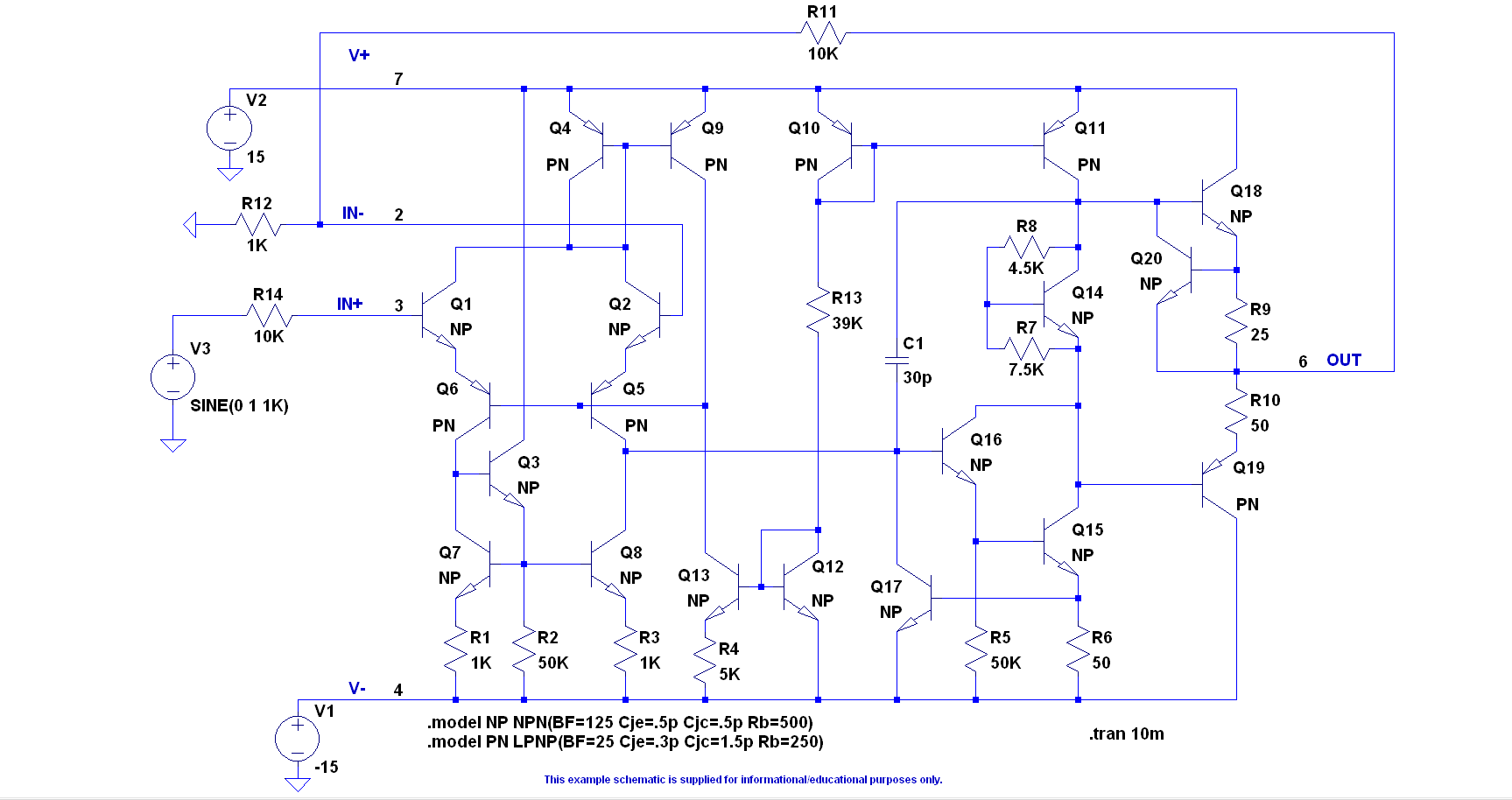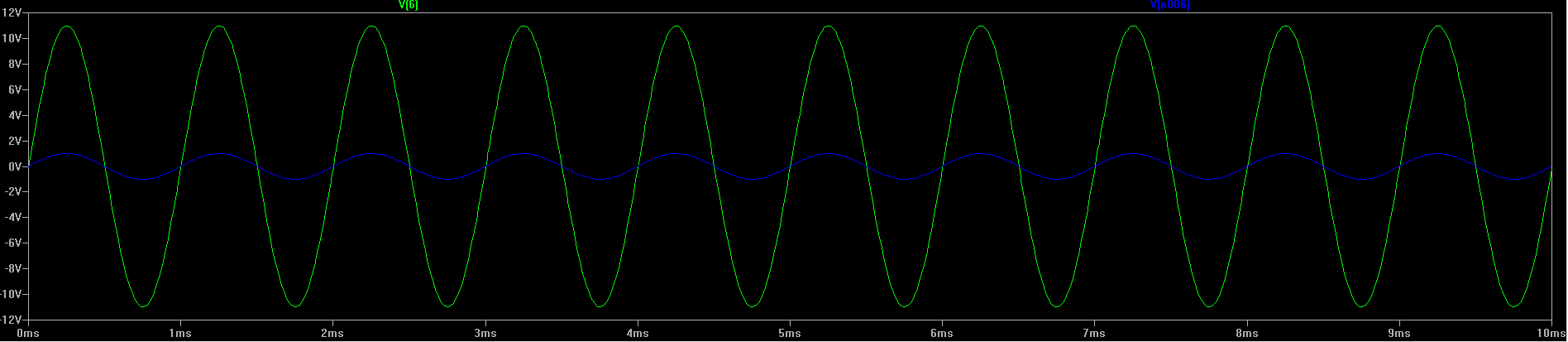Designing Analog Chips

What Makes Up an Opamp?

Operational-Amplifier Circuits• E
EricEverton November 27, 2015

Is it possible that the 2nd term in the equation for Ic(Q3) has an error in it?  The collector current of Q3 is equal to sum of the base current of Q7 or Q8 (2I/B) + the base current of Q7 not Q8.

I think the correct formula should be: Ic(Q3) = 2I/B + (Vbe(Q7)+IR2)/R1

Like.
• S
spetcavich November 30, 2015
Hm. I think thats another way of stating the same results. Vbe(Q8) = Vbe(Q7).
Like.
• B
Blaumeise July 26, 2017

Hi,

really good article, i appreciate it very much.

From my point of view Q18 and Q19 form a push pull stage and Q20 (instead of Q18) is current limitter.
it seems to be a little bit jumbled.

Like.